• 利用ARIMA季节模型预测
2022-04-04 15:28:51

## 目的：某公司业务具有明显的季节性波动，为了提前做好假日部署，需根据历史数据预测未来留存用户数量

1、数据准备

import pmdarima as pm
import pandas as pd
import matplotlib.pyplot as plt

#导入数据
data=pd.DataFrame(data)

#查看原始数据时序图
plt.rcParams['font.sans-serif'] = ['SimHei'] #用来正常显示中文标签
plt.rcParams['axes.unicode_minus'] = False #用来正常显示负号
data.plot()
plt.show()


2、查看数据基本情况

#查看原始数据自相关系数
from statsmodels.graphics.tsaplots import plot_acf
plot_acf(data).show()

dfoutput = pd.Series(dftest[0:4], index=['Test Statistic','p-value','Number of Lags Used','Number of Observations Used'])
for key,value in dftest.items():
dfoutput['Critical Value (%s)'%key] = value
print (dfoutput)



3、如果时间序列不平稳，需进行差分转换，得到差分之后的平稳序列再进行预测
（1）如果明确需一阶差分的话

#ADF检验结果说明原始序列不平稳，需进行差分，一阶 差分
D_data = data.diff(1).dropna()
D_data.columns = [u'liucun差分']
D_data.plot() #时序图
plt.show()
plot_acf(D_data).show() #自相关图

#对一阶差分后的序列进行单位根检验，P值小于0.05，一阶差分后的序列平稳
from statsmodels.graphics.tsaplots import plot_pacf
plot_pacf(D_data).show() #偏自相关图


(2)如果不明确需进一步定阶

#定好差分阶数后，对模型p，q定阶，可用aic或bic指标，ARIMA(data, (0, 0, 1)).fit().bic
from statsmodels.tsa.arima_model import ARIMA
pmax =int(len(data) / 5)    #一般阶数不超过 length /10
qmax = int(len(data) / 5)
aic_matrix = []
for p in range(pmax +1):
temp= []
for q in range(qmax+1):
try:
temp.append(ARIMA(data, (p, 1, q)).fit().aic)
except:
temp.append(None)
aic_matrix.append(temp)

aic_matrix = pd.DataFrame(aic_matrix)   #将其转换成Dataframe 数据结构
print(aic_matrix)

p,q = aic_matrix.stack().idxmin()   #先使用stack 展平， 然后使用 idxmin 找出最小值的位置
print(u'AIC 最小的p值 和 q 值：%s,%s' %(p,q))


4、定好阶后可带入ARIMA模型进行拟合

#找到是AIC/BIC最小的p,q参数，代入ARIMA模型中，进行拟合
from statsmodels.tsa.arima_model import ARIMA
print(ARIMA(data, (5,1,0)).fit().bic)
model=ARIMA(data, (5,1,0)).fit()
model.summary2()

#model.fittedvalues
fittedvalues = pd.Series(model.fittedvalues, copy=True)
plt.figure(figsize=(10, 6))
plt.plot(fittedvalues,label="forecast")
plt.plot(data,label="real")
plt.plot(D_data,label="diff")
plt.xlabel('month',fontsize=12,verticalalignment='top')
plt.ylabel('avgdau',fontsize=14,horizontalalignment='center')
plt.legend()
plt.show()



5、对拟合的模型效果进行评价

# 计算R平方值
import numpy as np
yhat =model.fittedvalues                        # or [p(z) for z in x]
ybar = np.sum(D_data[u'liucun差分'])/len(D_data[u'liucun差分'])          # or sum(y)/len(y)
ssreg = np.sum((D_data[u'liucun差分']-ybar)**2)   # or sum([ (yihat - ybar)**2 for yihat in yhat])
sstot = np.sum((D_data[u'liucun差分'] -yhat)**2)    # or sum([ (yi - ybar)**2 for yi in y])
R =1- (sstot/ssreg)
R


6、利用拟合出来的模型进行预测

#利用模型进行预测
model.forecast(7)   #为未来8个月进行预测， 返回预测结果， 标准误差， 和置信区间python
更多相关内容
• ## [Python][pmdarima] 季节性ARIMA模型学习

千次阅读 多人点赞 2020-08-16 12:48:32
前段时间参与了一个快消行业需求预测的项目。其中，用到了移动平均法、ARIMA、Xgboost等方法进行预测，现在打算总结一下ARIMA。 因为项目的销售数据属于私密数据，这里用网上找的一份案例数据用于展示。

# 背景

前段时间参与了一个快消行业需求预测的项目。其中，用到了移动平均法、ARIMA、Xgboost等方法进行预测，现在打算总结一下ARIMA。
因为项目的销售数据属于私密数据，这里用网上找的一份案例数据用于展示。
构建ARIMA模型可以用到statsmodels库和pmdarima库。我这里用的是pmdarima库，这个库有一个优点是能够自动地对ARIMA模型的参数进行自动确定。

## 1、建模步骤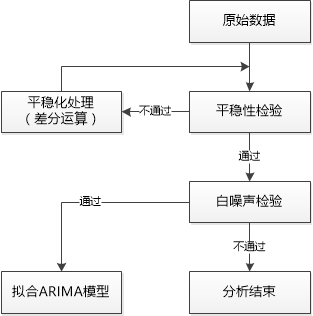## 2、ARIMA模型

• 首先， A R M A ( p , q ) \color{red}ARMA (p,q) 称为自回归移动平均模型，由 A R ( p ) AR(p) M A ( q ) MA(q) 模型结合而成，公式为： y t = μ + ∑ i = 1 p γ i y t − i + ϵ t + ∑ i = 1 q θ i ϵ t − i y_t=μ+\sum_{i=1}^p \gamma_i y_{t-i} +\epsilon_t + \sum_{i=1}^q \theta_i \epsilon_{t-i} 其中， μ μ 为常数项； p p 为自回归阶数； γ i \gamma_i θ i \theta_i 为待定系数； ϵ t \epsilon_t 为误差。
A R ( p ) AR(p) 描述当前值 y t y_t 与历史值之间的关系。
M A ( q ) MA(q) 关注的是自回归模型的误差项的累加，有效地消除预测中的随机波动。

有些时间序列可能呈现出季节性、周期性波动。这样的时间序列肯定产生于非平稳的随机过程，从而不可以直接套用 A R ( p ) AR(p) M A ( q ) MA(q) A R M A ( p , q ) ARMA(p,q) 之类的平稳随机过程来模拟。
对于非平稳的时间序列，应该将其平稳化。其中，差分化是最常用的平稳化方法。然后，再使用 A R ( p ) AR(p) M A ( q ) MA(q) A R M A ( p , q ) ARMA(p,q) 来模拟已经平稳化的时间序列。这就是所谓的差分自回归移动平均模型 A R I M A ( p , d , q ) \color{red}ARIMA(p,d,q) ，的 d d 是差分化的次数。

• 优缺点
优点： 模型十分简单，只需要内生变量而不需要借助其他外生变量。
缺点：（1）要求时序数据是平稳的，或者是通过差分化后是平稳的；（2）本质上只能捕捉线性关系，而不能捕捉非线性关系。

## 3、季节性ARIMA模型

A R I M A ( p , d , q ) ( P , D , Q ) m \color{red}ARIMA (p,d,q) (P,D,Q)_m 该模型需要提供的参数有如下两类：
（1）趋势参数
p p ：趋势自回归阶数。
d d ：趋势差分阶数。
q q ：趋势移动平均阶数。
（2）季节性参数
P P ：季节性自回归阶数。
D D ：季节性差分阶数。
Q Q ：季节性移动平均阶数。
m m ：单个季节期间的时间步数。

# 一、模块导入及数据读取

## 1、模块及数据

导入相关模块

from model.arimaModel import *   # 导入自定义的方法
import pandas as pd
import matplotlib.pyplot as plt
import pmdarima as pm
from statsmodels.graphics.tsaplots import plot_acf, plot_pacf  # 画图定阶
from statsmodels.stats.diagnostic import acorr_ljungbox        # 白噪声检验
import warnings
warnings.filterwarnings("ignore")  # 选择过滤警告


读取本地数据，需要数据可以留言。这里我用的数据是月份数据，如果有的数据集是日的数据，当发生太大波动性性很难发现趋势时，可以使用resample()进行每月重采样。

io = 'D:/PythonProject/ARIMA/AirPassengers.csv'
# 将字符串索引转换成时间格式
time_series.index = pd.to_datetime(time_series.index)
# 输出前5个数据，此时Date为时间索引
print("样本量为{}个".format(len(time_series)))## 2、季节性分析

# 时间序列图生成函数
def sequencePlot(data, sequencePlot_name):
data.plot(marker='o')
# 设置坐标轴标签
plt.xlabel("时间", fontsize=15)
plt.ylabel("销量", fontsize=15)
# 设置图表标题
plt.title("{}时间序列图".format(sequencePlot_name), fontsize=15)
# 图例位置
plt.legend(loc='best')
# 生成网格
plt.grid(linestyle='--')
plt.show()

orign_seqname = "原始"
sequencePlot(time_series, orign_seqname)


用matplotlib输出原始时间序列图如下。明显地，我们可以看到数据有上升的趋势，可以定性地判断该序列非平稳。另外，该时间序列有比较明显的季节性趋势，即基本上每一年数据都呈现先上升再下降。同时，可以使用seasonal_decompose()进行分析，将时间序列分解成长期趋势项(Trend)、季节性周期项(Seansonal)和残差项(Resid)这三部分。该方法的原理见这篇文章

from statsmodels.tsa.seasonal import seasonal_decompose

# 分解数据查看季节性   freq为周期
ts_decomposition = seasonal_decompose(time_series['Passengers'], freq=12)
ts_decomposition.plot()
plt.show()


由下子图，确实每年的数据呈现先升后降的特征。# 二、平稳性检验

ARIMA模型要求时间序列是平稳的。所谓平稳性，其基本思想是：决定过程特性的统计规律不随着时间的变化而变化。由平稳性的定义：对于一切 t , s t,s Y t Y_t Y s Y_s 的协方差对于时间的依赖之和时间间隔 ∣ t − s ∣ |t-s| 有关，而与实际的时刻t和s无关。因此，平稳过程可以简化符号，其中 C o v Cov 为自协方差函数， C o r r Corr 为自相关函数，记为： γ t = C o v ( Y t , Y t − k ) ， ρ k = C o r r ( Y t , Y t − k ) \gamma_t=Cov(Y_t,Y_{t-k}) ，\rho_k=Corr(Y_t,Y_{t-k}) 另外，平稳分为严平稳和宽平稳。定义如下：
（1）严平稳：特别地，如果对一切时滞 k k 和时点点 t 1 ， t 2 ， . . . ， t k t_1，t_2，...，t_k ，都有 Y t 1 , Y t 2 , . . . , Y t n Y_{t_1},Y_{t_2},...,Y_{t_n} Y t 1 − k , Y t 2 − k , . . . , Y t n − k Y_{t_1-k},Y_{t_2-k},...,Y_{t_n-k} 的联合分布相同，则称过程{ Y t Y_t }为严平稳的。
（2）宽平稳：满足1）均值函数在所有时间上恒为常数；2） γ t , t − k = γ 0 , k \gamma_{t,t-k}=\gamma_{0,k} ，对所有的时间 t t 和滞后 k k

详细的介绍可以看这篇博客
https://blog.csdn.net/FrankieHello/article/details/86766625/

## 2、Python实现

from statsmodels.tsa.stattools import adfuller                 # ADF检验

# 该函数参考了其他文章
def stableCheck(timeseries):
# 移动12期的均值和方差
rol_mean = timeseries.rolling(window=12).mean()
rol_std = timeseries.rolling(window=12).std()
# 绘图
fig = plt.figure(figsize=(12, 8))
orig = plt.plot(timeseries, color='blue', label='Original')
mean = plt.plot(rol_mean, color='red', label='Rolling Mean')
std = plt.plot(rol_std, color='black', label='Rolling Std')
plt.legend(loc='best')
plt.title('Rolling Mean & Standard Deviation')
plt.show()
print('Results of Dickey-Fuller Test:')
# 对检验结果进行语义描述
dfoutput = pd.Series(dftest[0:4], index=['Test Statistic', 'p-value', '#Lags Used', 'Number of Observations Used'])
for key, value in dftest.items():
dfoutput['Critical Value (%s)' % key] = value
print(dfoutput)

stableCheck_result1 = stableCheck(time_series)（1）这里将Test Statistic与1%、%5、%10不同程度拒绝原假设的统计值进行比较。当ADF Test result 同时小于 1%、5%、10%即说明非常好地拒绝该假设。本数据中，0.815369并没有都小于三个level的统计值，所以判断为该时间序列time series非平稳。
（2）观察p-value，是否非常接近于0。这里p-value约为0.99>0.05，因此不能拒绝原假设。# 三、序列平稳化

## 差分法

对于非平稳时间序列，可以通过d阶差分运算使变成平稳序列。从统计学角度来讲，只有平稳性的时间序列才能避免“伪回归”的存在，才有经济意义。

# 差分处理非平稳序列，先进行一阶差分
time_series_diff1 = time_series.diff(1).dropna()
# 在一阶差分基础上进行季节性差分差分
time_series_diff2 = time_series_diff1.diff(12).dropna()
stableCheck_result2 = stableCheck(time_series_diff2)


结果如下，p-value小于0.05，且Test Statistic远小于Critical Value (5%)的值，可以有95%的概率认为时间序列平稳。
因此，我们可以确定季节性ARIMA模型的 d = 1 ， D = 1 ， m = 12 d=1，D=1，m=12# 四、白噪声检验

接下来，对d阶差分后的的平稳序列进行白噪声检验，若该平稳序列为非白噪声序列，则可以进行第五步。

## 1、白噪声定义

对于一序列 e 1 , e 2 , e 3 , . . . , e t e_1,e_2,e_3,...,e_t ，若满足： E ( e t ) = 0 E(e_t)=0 V a r ( e t ) = σ 2 Var(e_t)=σ^2 C o v ( e t , e t + k ) = 0 （ k ≠ 0 ） Cov(e_t,e_{t+k})=0 （k≠0） 那么该序列是一个白噪声序列。

详细的介绍可以看这篇博客
https://www.cnblogs.com/travelcat/p/11400307.html

## 2、Ljung-Box检验

Ljung-Box检验即LB检验，是时间序列分析中检验序列自相关性的方法。LB检验的Q统计量为：
Q ( m ) = T ( T + 2 ) ∑ l = 1 m ρ ^ l 2 T − l Q(m)=T(T+2) \sum_{l=1}^m \frac{\hat{ρ}_l^2}{T-l} \quad       用来检验 m m 阶滞后范围内序列的自相关性是否显著，或序列是否为白噪声，Q统计量服从自由度为 m m 的卡方分布。

## 3、Python实现

def whiteNoiseCheck(data):
result = acorr_ljungbox(data, lags=1)
temp = result
print('白噪声检验结果：', result)
# 如果temp小于0.05，则可以以95%的概率拒绝原假设，认为该序列为非白噪声序列；否则，为白噪声序列，认为没有分析意义
print(temp)
return result

ifwhiteNoise = whiteNoiseCheck(time_series_diff2)


检验结果如下：返回的是统计量和p-value，其中，延迟1阶的p-value约为0.00033<0.05，可以以95%的概率拒绝原假设，认为该序列为非白噪声序列。# 五、时间序列定阶

def draw_acf(data):
# 利用ACF判断模型阶数
plot_acf(data)
plt.title("序列自相关图(ACF)")
plt.show()

def draw_pacf(data):
# 利用PACF判断模型阶数
plot_pacf(data)
plt.title("序列偏自相关图(PACF)")
plt.show()

def draw_acf_pacf(data):
f = plt.figure(facecolor='white')
# 构建第一个图
# 把x轴的刻度间隔设置为1，并存在变量里
x_major_locator = MultipleLocator(1)
plot_acf(data,  ax=ax1)
# 构建第二个图
plot_pacf(data, ax=ax2)
# 把x轴的主刻度设置为1的倍数
ax1.xaxis.set_major_locator(x_major_locator)
ax2.xaxis.set_major_locator(x_major_locator)
plt.show()

draw_acf_pacf(time_series_diff2)


具体怎么对季节性ARIMA模型进行定阶数可以看这里
（1）确定 d d D D 的阶数：当对原序列进行了 d d 阶差分和 l a g lag m m D D 阶差分后序列为平稳序列，则可以确定 d d D D m m 的值。
（2）确定 p p q q P P Q Q 的阶数：1）首先对平稳化后的时间序列绘制ACF和PACF图；2）然后，可以通过观察季节性 l a g lag 处的拖尾/截尾情况来确定 P , Q P,Q 的值；3）观察短期非季节性 l a g lag 处的拖尾/截尾情况来确定 p , q p,q 的值。
之前已经在步骤三处确定了 d d D D m m 。对于剩下的参数，将依据如下的ACF和PACF图确定。下图的横坐标 l a g lag 是以月份为单位。
非季节性部分：对于 p p ，在 l a g = 1 lag=1 后，ACF图拖尾，PACF图截尾。同样地，对于 q q ，在 l a g = 1 lag=1 后，ACF图截尾，PACF图拖尾。
季节性部分 P ， Q P，Q 的确定和非季节性一样，不过需要记得滞后的间隔为12。

【补充参考】
AR模型：自相关系数拖尾，偏自相关系数截尾；
MA模型：自相关系数截尾，偏自相关函数拖尾；
ARMA模型：自相关函数和偏自相关函数均拖尾。# 六、构建ARIMA模型及预测

这里使用了pmdarima.auto_arima()方法。这个方法可以帮助我们自动确定 A R I M A ( p , d , q ) ( P , D , Q ) m ARIMA(p,d,q)(P,D,Q)_m 的参数，也就是可以直接跳过上述的步骤2~5，直接输入数据，设置auto_arima()中的参数则可。
之前我们是通过观察ACF、PACF图的拖尾截尾现象来定阶，但是这样可能不准确。实际上，往往需要结合图像拟合多个模型，通过模型的AIC、BIC值以及残差分析结果来选择合适的模型。

## 1、构建模型

import pmdarima as pm


将数据分为训练集data_train和测试集data_test 。

split_point = int(len(time_series) * 0.85)
# 确定训练集/测试集
data_train, data_test = time_series[0:split_point], time_series[split_point:len(time_series)]
# 使用训练集的数据来拟合模型
built_arimamodel = pm.auto_arima(data_train,
start_p=0,   # p最小值
start_q=0,   # q最小值
max_p=5,     # p最大值
max_q=5,     # q最大值
m=12,        # 季节性周期长度，当m=1时则不考虑季节性
d=None,      # 通过函数来计算d
seasonal=True, start_P=0, D=1, trace=True,
error_action='ignore', suppress_warnings=True,
stepwise=False  # stepwise为False则不进行完全组合遍历
)
print(built_arimamodel.summary())


相关结果如下，从Model可以看出确定的参数与之前确定的参数大致相同。## 2、模型识别

built_arimamodel.plot_diagnostics()
plt.show()## 3、需求预测

# 进行多步预测,再与测试集的数据进行比较
pred_list = []
for index, row in data_test.iterrows():
# 输出索引，值
# print(index, row)
pred_list += [built_arimamodel.predict(n_periods=1)]
# 更新模型，model.update()函数，不断用新观测到的 value 更新模型
built_arimamodel.update(row)
# 预测时间序列以外未来的一次
predict_f1 = built_arimamodel.predict(n_periods=1)
print('未来一期的预测需求为：', predict_f1)


## 3、结果评估

对预测结果进行评价，指标解释看这

# ARIMA模型评价
def forecast_accuracy(forecast, actual):
mape = np.mean(np.abs(forecast - actual)/np.abs(actual))
me = np.mean(forecast - actual)
mae = np.mean(np.abs(forecast - actual))
mpe = np.mean((forecast - actual)/actual)
# rmse = np.mean((forecast - actual)**2)**0.5    # RMSE
rmse_1 = np.sqrt(sum((forecast - actual) ** 2) / actual.size)
corr = np.corrcoef(forecast, actual)[0, 1]
mins = np.amin(np.hstack([forecast[:, None], actual[:, None]]), axis=1)
maxs = np.amax(np.hstack([forecast[:, None], actual[:, None]]), axis=1)
minmax = 1 - np.mean(mins/maxs)
return ({'mape': mape,
'me': me,
'mae': mae,
'mpe': mpe,
'rmse': rmse_1,
'corr': corr,
'minmax': minmax
})

# 画图观测实际与测试的对比
test_predict = data_test.copy()
for x in range(len(test_predict)):
test_predict.iloc[x] = pred_list[x]
# 模型评价
eval_result = forecast_accuracy(test_predict.values, data_test.values)
print('模型评价结果\n', eval_result)
# 画图显示
plt.plot(origin_timeseries, 'b-', lw=1, label='True Data', marker='*')
plt.plot(test_predict, 'r-', lw=2, label='Prediction', marker='o')
plt.title('RMSE:{}'.format(eval_result['rmse']))
plt.legend(loc='best')
plt.grid()  # 生成网格
plt.show()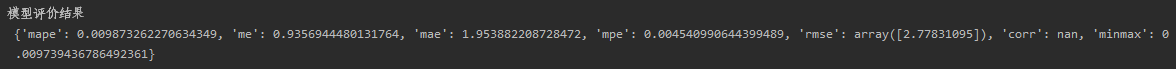红色的点为预测结果，蓝色的点为原来的数据。
RMSE约为16.4，均方根误差（Root Mean Square Error，RMSE）是预测值与真实值偏差的平方与观测次数n比值的平方根指标，衡量观测值与真实值之间的偏差。一般是越小越好，但是实际上需要进行结果对比才能判断。# 七、小结

在做项目时候，发现ARIMA模型对数据的要求还是挺高的。其中，数据量太少不行，当时做的时候发现一些门店的数据量太少，而且只有一年左右的跨度，很难发现一些周期规律。另外就是对平稳性的要求。
目前对时间序列分析还是初学阶段，很多地方的解释不够深入，后续还需要继续学习、完善。

展开全文机器学习 python

# 前言

时间：19/04/25，天气晴，心情愉悦。

# ARIMA模型简介

具有如下结构的模型称为求和自回归移动平均(Autoregressive Integrated Moving Average)，简记为ARIMA(p,d,q)模型：式中：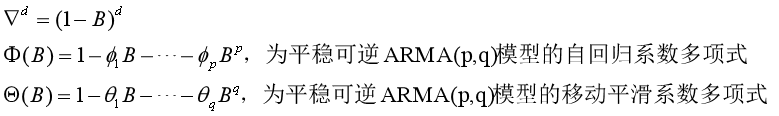ARIMA模型的数学原理和公式我就不一一解释了，如果有不懂的地方可以参考ARIMA模型，在本篇中我们主要探讨如何用Python对时序进行分析建模。

# Python实现ARIMA模型预测

## 数据的获取与准备

如某市1995-2003年各月的工业生产总值如下表所示，试对1995-2002年数据建模，2003年的数据留做检验模型的预测结果。我们在获取数据时，可能会因为一些客观因素（比如一些表格编辑软件的自动将编码转换UTF-8，默认的时间格式规范不一致，例如19/04/25可能会转换成04/25/19，还有就是本身数据就存在缺失、不合理等问题）导致了数据的异常或缺失，然而在机器学习里，数据的好坏直接决定了模型结果的好坏，所以对数据的预处理必不可少。在我们这个实验数据中，数据并没有出现缺失以及不符逻辑的情况，所以我们可以直接将其作为ARIMA模型的输入数据。（如果数据出现缺失不合理等现象，可以选择性参考 https://blog.csdn.net/zhangyonggang886/article/details/80901290

Python3：

import csv
dataSet = [] # 训练数据集
dateSet = [] # 训练年份集
for data in data_files:
if data == '200301': # 将csv文件中验证数据去除
break
if data != 'date':
dateSet.append(data)
dataSet.append(float(data))


## 绘制1995-2002年时间序列趋势图

显示一行一行的数据是难以观察数据的趋势走向，这样不利于我们观测数据之间的相互联系，因此画出趋势图有利于数据分析。

Python3：

# 利用Pandas库来绘制时间序列趋势图
import matplotlib.pyplot as plt
import pandas as pd
df = pd.DataFrame(dataSet,index=dateSet,columns=['real value'])
df.plot()
plt.show()


result：①为什么要去均值化？做数据的去均值化其实就是对数据进行标准化，在很多情况下，我们对数据本身大小并不感兴趣，我们感兴趣的是数据之间的联系，去均值化并不会影响这种联系，因为去均值化后得到的时间序列趋势和没有去均值化得到的时间序列趋势是一样的。
Python3：

# 对时间序列进行去均值化
mean = sum(dataSet)/len(dataSet) # 计算均值
dataSet_kill_mean = [data - mean for data in dataSet] # 得到去均值后的序列


②序列平稳是做ARIMA回归的前提条件，所以我们得到的序列必须是平稳的。观测上面的序列趋势图，我们可以很明显的发现数据有逐渐上升的趋势，因此我们判断该段时间序列为非平稳序列。为了得到平稳的时间序列，我们要做差分，因为差分目的是使变量序列平稳。
Python3：

# 进行一阶差分并绘制趋势图
df_kill_mean = pd.DataFrame(dataSet_kill_mean,index=dateSet,columns=['kill mean value'])
df_kill_mean_1 = dataSet_kill_mean.diff(1).dropna()
df_kill_mean_1.plot()
plt.show()


result：Python3：

import statsmodels.tsa.stattools as ts
>>> (-3.3635866783352, 0.012264631212932628, 12, 82, {'1%': -3.512738056978279, '5%': -2.8974898650628984, '10%': -2.585948732897085}, 237.8874357065477)


如何观察上面的统计结果来判断序列是否为平稳呢？

2. P-value是否非常接近0。本结果中，P-value 为 0.012264631212932628 < 0.05并接近0。

由此，通过检验观测值，我们认为上述一阶差分后得到的数据满足平稳性检验要求。（一阶差分后的序列平稳性不太好，有可能通不过白噪声检验，在这里忽略白噪声检验环节，若白噪声检验得到的P值大于0.05，那么我们就得对时间序列进行二阶差分）

## 绘制自相关函数以及偏相关函数图确定参数p、q

参数确认规则：（已知序列为一阶差分）
① 当偏自相关函数呈现p阶拖尾，自相关函数呈现q阶拖尾时，我们可以选用模型ARIMA（p，1，q）
② 当偏自相关函数呈现拖尾，自相关函数呈现q阶截尾时，我们可以选用模型MA（q）
③ 当偏自相关函数呈现p阶截尾，自相关函数呈现拖尾时，我们可以选用模型AR（p）
（备注：如果想要深入了解如何通过PACF和ACF函数图确定模型以及参数，可以参考《ARMA模型的自相关函数和偏自相关函数图谱》链接：https://pan.baidu.com/s/1JqkIIh1gdHqjp9uwwGC87A 提取码：1koe （如果链接失效，联系我重新发链接）

Python3：

from statsmodels.graphics.tsaplots import plot_acf, plot_pacf
# 绘制自相关图
plot_acf(df_kill_mean_1,lags=24).show() # 其中lags参数是指横坐标最大取值
# 绘制偏相关图
plot_pacf(df_kill_mean_1,lags=24).show()
plt.show()


rusult：我们观测ACF函数图和PACF函数图，我们发现并没有呈现很好的拖尾或截尾情况。出现这个情况的原因有很多种情况，我们观测函数图发现，每隔一个周期，ACF和PACF都出现“尖峰“，例如上述图每隔12个月出现一个"尖峰"，由此我们可以很容易的判断，该序列可能存在季节性影响的因素（其实由开始的序列趋势图我们也可以看出序列存在季节性影响）。
我们可以通过分解的方式将时序数据分离成不同的成分，它主要将时序数据分离为Trend（成长趋势）、seasonal（季节性趋势）、Residuals（随机成分）。然后我们分别对这三个分离的序列进行ARIMA建模得到较好的模型，最后再将模型相加便可以得到最后的ARIMA模型。

Python3：

from statsmodels.tsa.seasonal import seasonal_decompose
decomposition = seasonal_decompose(df_kill_mean_1, freq=12)
trend = decomposition.trend   # 趋势部分
seasonal = decomposition.seasonal # 季节性部分
residual = decomposition.resid # 残留部分
decomposition.plot()


result：这种分解建模的方式有些复杂，接下来我们采用季节性时间序列来对上述具有明显季节性的序列进行建模。

## 建立季节性时间序列模型ARIMA(k,D,m)S×(p,d,q)

我们称ARIMA(k,D,m)S×(p,d,q)为乘积季节模型，也可以写成ARIMA(p,d,q)(k,D,m)S模型，其中S为季节性周期。如果将模型中的AR因子和MA因子分别展开，可以得到类似的ARMA(kS+p,mS+q)的模型。当我们考虑用ARIMA(p,d,q)(k,D,m)S模型的时候，我们需要优化感兴趣度量的是ARIMA(p,d,q)(k,D,m)s各个参数的值。接下来，我们将使用“网格搜索”来迭代地探索参数的不同组合。 对于参数的每个组合，我们使用statsmodels模块的SARIMAX()函数拟合一个新的季节性ARIMA模型，并评估其整体质量。

Python3：

# 这段代码借鉴了其他博文的做法
import itertools
p = q = range(0, 2) # p、q一般取值不超过2
d = range(1,2)
pdq = list(itertools.product(p, d, q))
seasonal_pdq = [(x, x, x, 12) for x in list(itertools.product(p, d, q))]
warnings.filterwarnings("ignore") # 忽略警告信息
for param in pdq:
for param_seasonal in seasonal_pdq:
try:
mod = sm.tsa.statespace.SARIMAX(df_mean_1,
order=param,
seasonal_order=param_seasonal,
enforce_stationarity=False,
enforce_invertibility=False)

results = mod.fit()
print('ARIMA{}x{} - AIC:{}'.format(param, param_seasonal, results.aic))
except:
continue
>>>
ARIMA(0, 1, 0)x(0, 1, 0, 12) - AIC:322.6198841487564
ARIMA(0, 1, 0)x(0, 1, 1, 12) - AIC:270.20449054798723
ARIMA(0, 1, 0)x(1, 1, 0, 12) - AIC:279.7073077170952
ARIMA(0, 1, 0)x(1, 1, 1, 12) - AIC:272.20429383065317
ARIMA(0, 1, 1)x(0, 1, 0, 12) - AIC:248.2004574618413
ARIMA(0, 1, 1)x(0, 1, 1, 12) - AIC:209.46795258585362
ARIMA(0, 1, 1)x(1, 1, 0, 12) - AIC:219.76010995507397
ARIMA(0, 1, 1)x(1, 1, 1, 12) - AIC:213.63266407486356
ARIMA(1, 1, 0)x(0, 1, 0, 12) - AIC:292.5090537220262
ARIMA(1, 1, 0)x(0, 1, 1, 12) - AIC:250.76816771015618
ARIMA(1, 1, 0)x(1, 1, 0, 12) - AIC:251.43830162843227
ARIMA(1, 1, 0)x(1, 1, 1, 12) - AIC:251.1402407735711
ARIMA(1, 1, 1)x(0, 1, 0, 12) - AIC:243.34941237032209
ARIMA(1, 1, 1)x(0, 1, 1, 12) - AIC:206.44248559031112
ARIMA(1, 1, 1)x(1, 1, 0, 12) - AIC:211.64269806160195
ARIMA(1, 1, 1)x(1, 1, 1, 12) - AIC:210.80404367428187


在这里，我们是通过最佳准则函数法来确定具体的参数值的，模型选择的AIC准则越小，我们就大概可以认为该模型越优。通过上述结果我们可以容易得到ARIMA(0, 1, 1)(0, 1, 1,)12模型相对最优。因此我们选择ARIMA(0, 1, 1)(0, 1, 1,)12模型作为我们预测时间序列的最佳模型。

## 模型预测

我们使用我们选取的最佳模型进行预测。

Python3：

import statsmodels.api as sm
df = pd.DataFrame(dataSet,index=dateSet,columns=['real value']) # 原始时间序列
mod = sm.tsa.statespace.SARIMAX(df,order=(0,1,1),seasonal_order=(1, 1, 1, 12),enforce_stationarity=False,enforce_invertibility=False)
result = mod.fit()
predict_sunspots = result.forecast(12)
forcast = np.array(predict_sunspots[:]).reshape(-1)
print(forcast)
>>>
[19.42912085 17.40614606 21.53290186 22.27891    23.06918964 23.69105707
22.54036142 23.49219397 24.56108863 24.69965562 26.02344756 26.41517033]


## 模型指标MAPE计算预测值和真实值得MAPE指标，得到MAPE指标值为3.14%，通过这个指标我们可以认为该模型是一个好模型。

## 预测值和真实值趋势对比图# 结尾

这是我第一次建立季节性ARIMA模型，如果中间出现错误或者有不合理的地方，还望各位海涵并指正出来，大家一起学习就是要相互纠错，只有这样大家才可以在学习上相互收益、相互进步。同时我也希望这篇文章可以给苦恼于如何用Python建立时间序列模型的朋友指明一条明路。

展开全文Python
• ## 季节性ARIMA模型【R语言】

万次阅读 多人点赞 2018-10-18 16:31:43
季节性ARIMA模型可以预测含有季节性，趋势性的时间序列。他的形式如下 这里m是每一季节的周期值。季节项与非季节项的模型非常相近。但是季节项中包含了季节周期性。例如对于ARIMA(1,1,1)(1,1,1)4模型能够写成： ...

季节性的ARIMA模型可以预测含有季节性，趋势性的时间序列。他的形式如下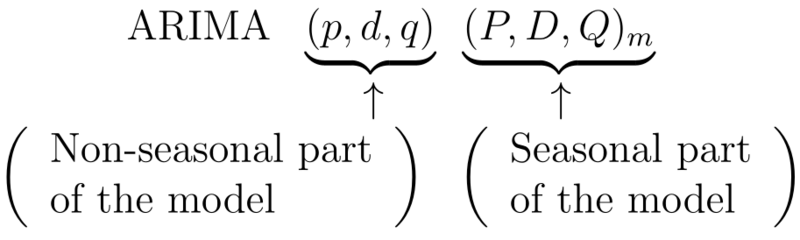这里m是每一季节的周期值。季节项与非季节项的模型非常相近。但是季节项中包含了季节周期性。例如对于ARIMA(1,1,1)(1,1,1)4模型能够写成：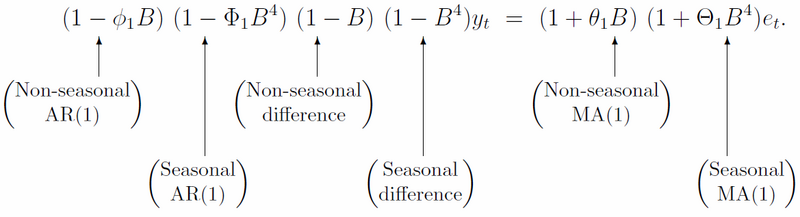ACF与PACF
对于AR与MA模型的季节项，我们将会在ACF和PACF的lags上看到差异。例如，ARIMA(0,0,0)(0,0,1)12模型，我们将会在ACF的lag12处看到一个spike(长钉，即非零显著的形象描述)，而在其他地方看不到spike。PACF将会在具有周期性的位置出现指数衰减，即lags 12，24，36…类似的，一个ARIMA(0,0,0)(1,0,0)12模型则会显示出在ACF图的周期性位置显示出指数衰减，而在PACF图中的lag12处看到一个spike。我以中国人民大学统计学院易丹辉教授在时间序列课堂上给出的数据book19为例进行分析，这里用到的软件是R，课上易丹辉教授用的是EViews6.0。
R程序:
data=ts(data[,2],frequency=12,start=c(1990,1)) #[,2]是只读取第2列数据，start是在数据上增加起始时间，frequency是增加周期，ts为转换成ts格式。
library(forecast) #载入程序包forecast，如果提示之前没有安装，则用install.package(“forecast”)进行安装，再载入forecast包。
tsdisplay(data,xlab=“year”,ylab=“Retail index”) #显示自相关系数ACF,偏自相关系数PACF,与数据原始图像。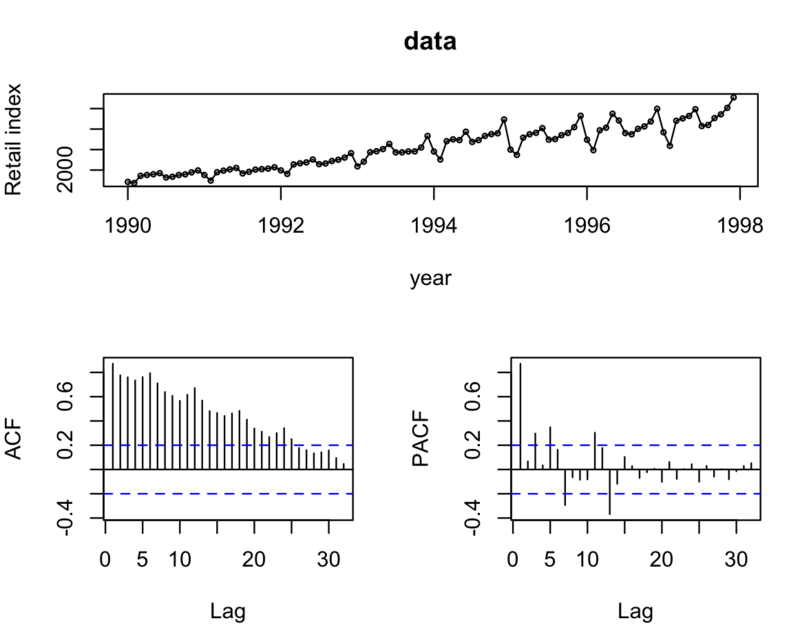data_d1=diff(data,1)
从上图可以看出自相关系数呈现拖尾性，即序列Yt与之前的时刻有强烈的相关性，而且相关项的范围是比较大的，说明序列本身有趋势，为了消除这种趋势我们取每一项与前一项的差。
tsdisplay(data_d1,xlab=“year”,ylab=“Retail index”)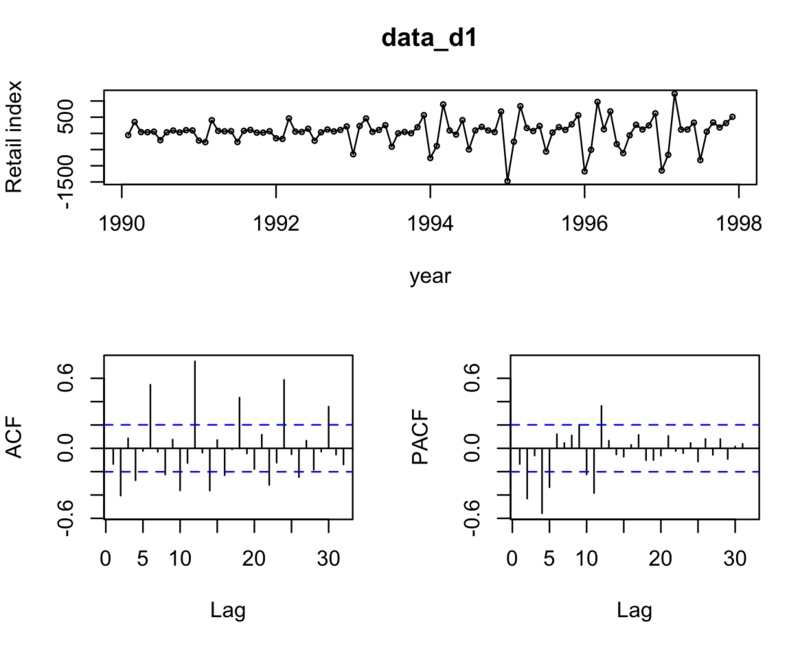可以看出来，趋势基本消除了。序列较平稳。而PACF在季节性周期lags12处有spike，因此进一步做季节差分。
data_d1D1=diff(data_diff1,12)
tsdisplay(data_d1D1,xlab=“year”,ylab=“Retail index”)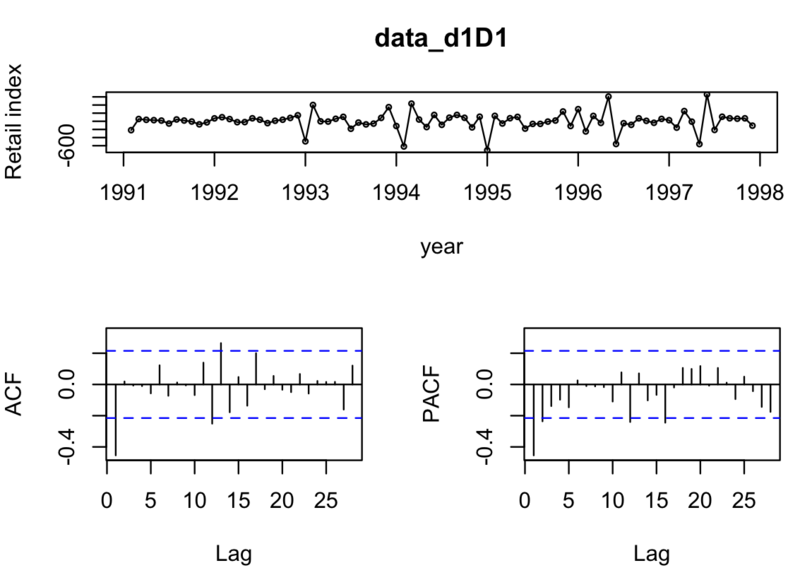所以对于非季节项，我们只做了一阶非季节差分，故d=1,从PACF看出p=2或3，从ACF看出q=1
对于季节项，我们也只做了一阶季节差分，故D=1，再看在lag12，24处看是否有spike，从PACF看出P=1，从ACF看出Q=1
从而选择模型一共有：Arima(2,1,1)(1,1,1)和Arima(3,1,1)(1,1,1)

fit1 <- Arima(data, order=c(2,1,1),seasonal=c(1,1,1))
fit1 #显示AICc=1110.09
tsdisplay(residuals(fit1)) #显示残差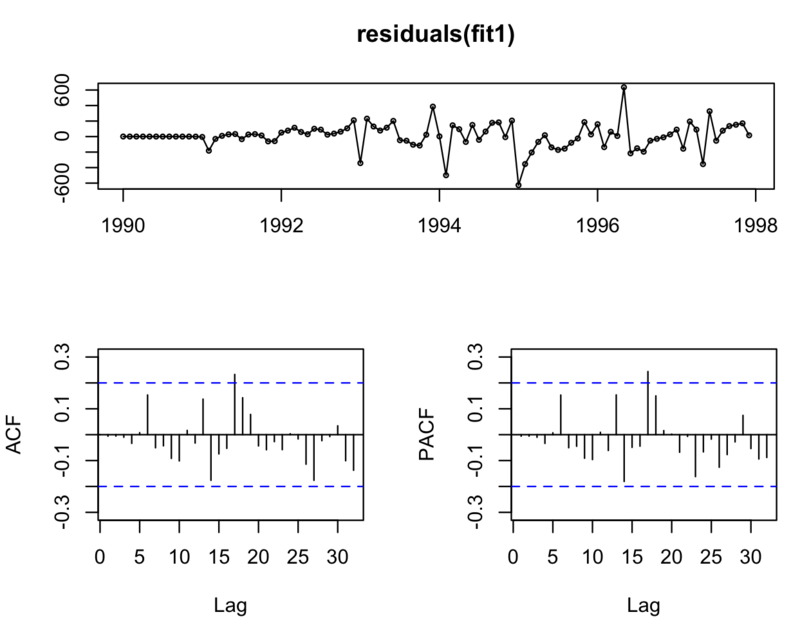fit2 <- Arima(data, order=c(3,1,1),seasonal=c(1,1,1))
fit2 #显示 AICc=1112.3
tsdisplay(residuals(fit1)) #显示残差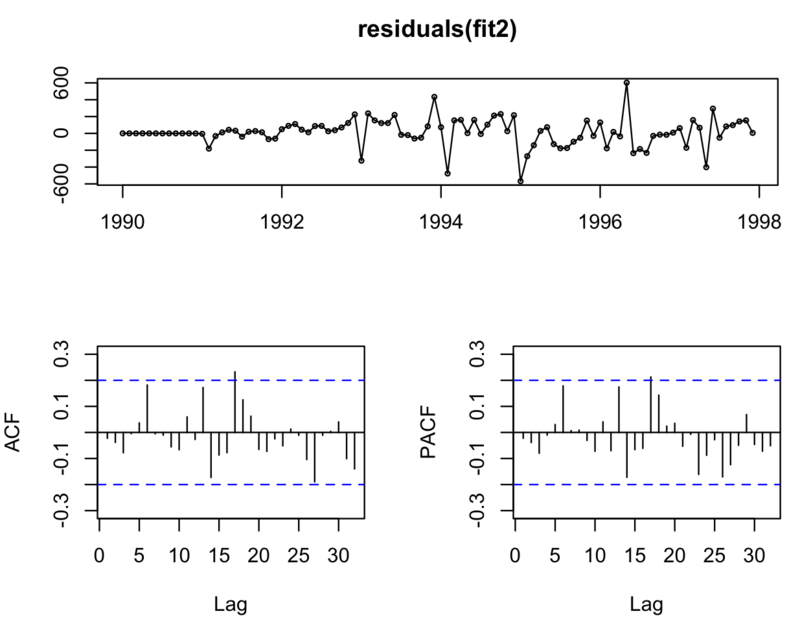plot(forecast(fit1,h=12)) #选择AICc值小的，输出我们最终的预测结果～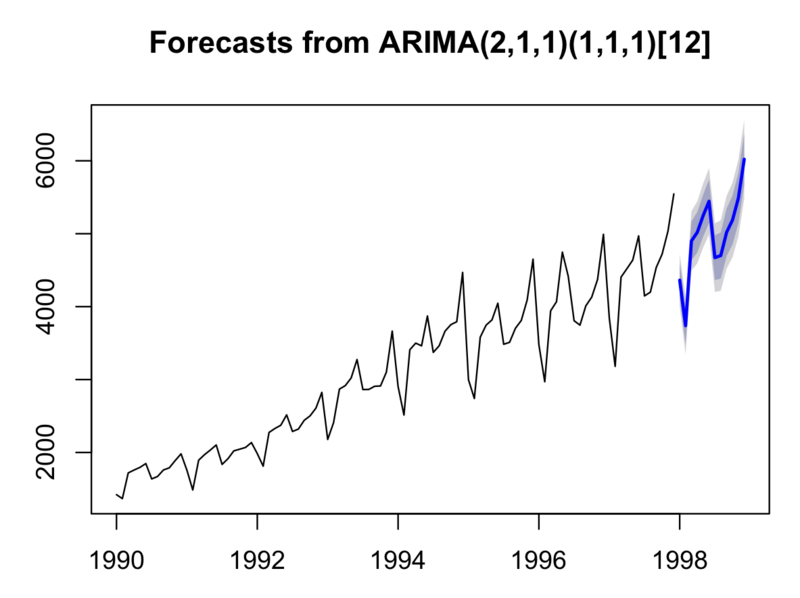还有一个更加傻瓜的做法就是使用auto.arima函数自动定p,d,q,P,D,Q等参数。
arima1<-auto.arima(data,trace=T)
如下图：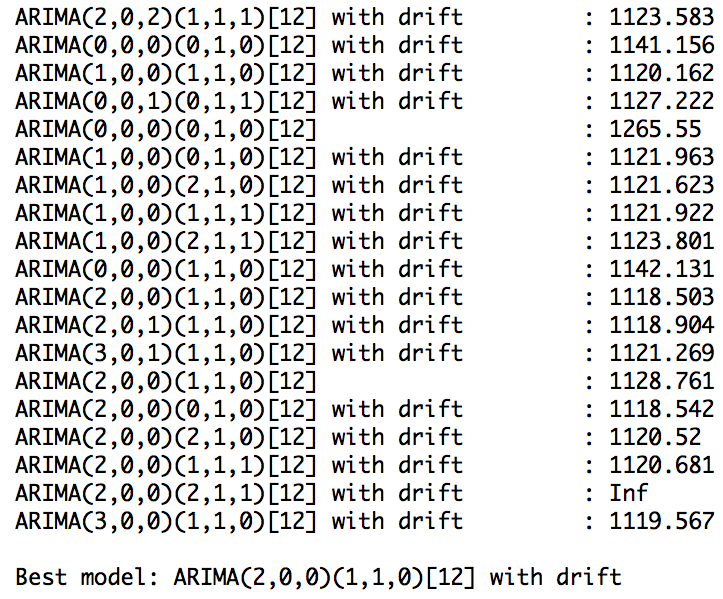with drift代表有趋势，所以最终模型可以加上d=1去除趋势。
最终的模型可以是：
Arima(2,1,0)(1,1,0)
其AICc=1107.86

plot(forecast(Arima(data,order=c(2,1,0),seasonal=c(1,1,0)),h=12))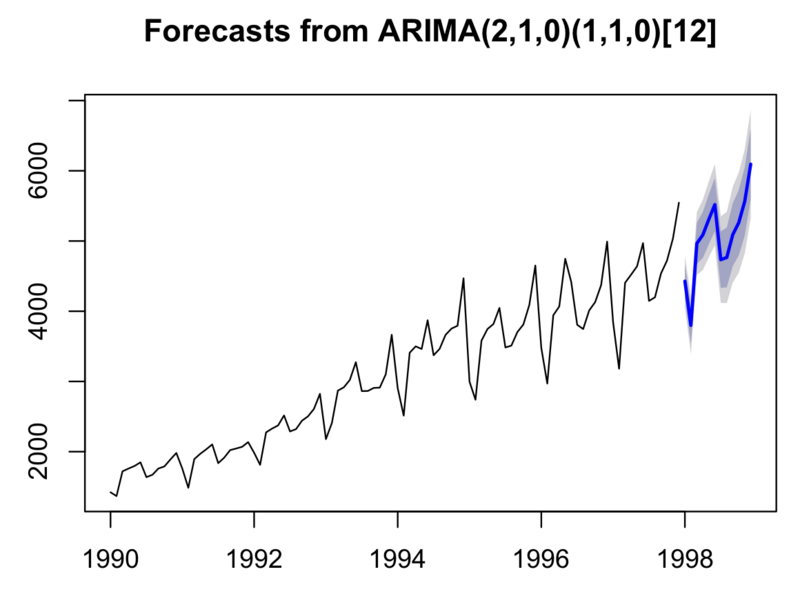这个结果是各个模型中AICc最小的。

展开全文R语言
• ## ARIMA模型的季节模型

万次阅读 多人点赞 2017-06-26 20:02:10
ARIMA模型可以对具有季节效应的序列建模，根据季节效应提取的难易程度可以分为简单季节模型与乘积季节模型。
• SARIMAX (seasonal autoregressive integrated moving average with exogenous regressor)是一种常见的时间序列预测方法，可以分为趋势部分和周期部分；每个部分又可以分为自回归、差分和平滑部分。 趋势稳定...时间序列
• arima模型怎么拟合 什么是ARIMA？ (What is ARIMA?) ARIMA models are one of the most classic and most widely used statistical forecasting techniques when dealing with univariate time series. It basically...python 机器学习 人工智能 tensorflow 算法...